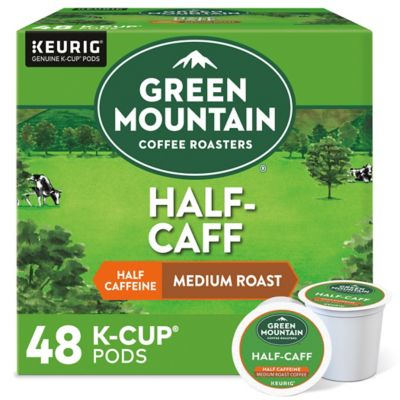# Coffee

## Coffee

Showing 1 - 24 of 85 products
Earn 3x points
Earn 3x points
Earn 3x points
Earn 3x points
Green Mountain Coffee®
1854 reviews
\$25.00(\$0.52 per count)Was \$32.99

Excluded from coupons

Sale!

Ready tomorrow for pickup at Dulles

Delivered today when you order by 4pmEarn 3x points
Earn 3x points
Earn 3x points
Earn 3x points
Earn 3x points
Earn 3x points
Earn 3x points
Earn 3x points
Best Seller
Earn 3x points
Earn 3x points
Earn 3x points
Earn 3x points
Earn 3x points
Earn 3x points
Earn 3x points
Earn 3x points
Earn 3x points
Earn 3x points
Best Seller
Earn 3x points
Earn 3x points
Top Rated
Earn 3x points
Earn 3x points
Earn 3x points
Earn 3x points
Earn 3x points
Earn 3x points
Earn 3x points
Earn 3x points
Earn 3x points
Earn 3x points
Earn 3x points
Earn 3x points
Earn 3x points
Earn 3x points
Earn 3x points
Earn 3x points
Earn 3x points
Earn 3x points
Earn 3x points
Earn 3x points